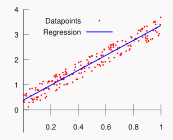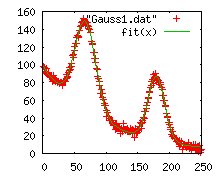## StatisticsMany practical modeling problems have an aspect that involves statistics. In this section we mention some documents that can help you incorporating statistical techniques in your projects. Many regression problems can also be formulated as optimization problems. However, specialized solvers may provide better performance and give more detailed output such as standard errors, covariance matrices, p-values etc. A good example is Linear Regression (OLS). There is no good way to formulate a numerically stable linear regression problem in GAMS, so for this problem the specialized LS solver can help.

### GAMS/LS: a Linear Regression Solver for GAMSThere is no good way to express a linear regression model in GAMS. An explicit minimization problem will be non-linear as it needs to express a sum of squares. Alternatively, a linear formulation using the normal equations (X'X)b=X'y will introduce numerical instability (see example longley2.gms below).

Therefore we have introduced a compact notation where we replace the objective by a dummy equation: the solver will implicitly understand that we need to minimize the sum of squared residuals. The GAMS/LS solver will understand this notation and can apply a stable QR decomposition to solve the overdetermined model quickly and accurately.

 The basic model will look like: ```sumsq.. sse =n= 0; fit(i).. data(i,'y') =e= b0 + b1*data(i,'x'); option lp = ls; model leastsq /fit,sumsq/; solve leastsq using lp minimizing sse; ```
Here sse is a free variable that will hold the sum of squared residuals after solving the model. The variables b0 and b1 are the statistical coefficients to be estimated. On return the levels are the estimates and the marginals are the standard errors.

The fit equations describe the equation to be fitted.

Examples

### GAMS/NLS: a Nonlinear Regression Solver for GAMSIn some cases we have a nonlinear statistical model to estimate: y=f(X,θ). In this case we cannot use linear algebra to find the minimizer but need to employ a numerical minimization technique. The GAMS/NLS uses NL2SOL. In addition it can use a starting point found by any of the GAMS NLP solvers. A major advantage of using GAMS is that the modeler does not have to provide derivatives.

### Bootstrap code for forming confidence intervals in Max Entropy estimation

Maximum entropy estimation has become an important tool when few observations are available. Confidence intervals can be formed using the bootstrap or resampling approach. The percentile method is used to estimate the confidence intervals. We use the RANK utility for this.

### Retrieve Hessian to form variances in maximum likelihood estimationGAMS 22.8 has a few facilities to retrieve the Hessian. These examples show how this can be used to estimate variance and standard errors in some maximum likelihood estimation applications.

### DocumentsHere are some documents that deal with statistics in a GAMS environment. They are all in PDF format. In many cases the documents contains download links to make it easier to retrieve the models.
 LS: a linear regression solver: run linear regression (OLS) from GAMS. NLS: a nonlinear regression solver: run nonlinear regression from GAMS. For difficult problems the GAMS solvers can provide a (near) optimal starting point. Different regression methods formulated as optimization problems. Random number generation. Data envelopment analysis. Special functions (related to statistics) in GAMS.

RegressionSolvers.zip

This zip file contains the GAMS/LS and GAMS/NLS regression solvers (Windows 32 bit). Note that under GAMS 22.8 LS is included. For earlier GAMS releases or for GAMS/NLS you can use this download.